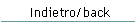ConsumptionThe following are some examples of electrical consumption over 24 hours, in two different scenarios: boat at anchor (most electronics not in use, anchor light at night)   boat sailing (most electronics + autopilot are in use, nav-lights at night) The first example refers to a "typical" configuration, while the second assumes that measures have been taken to reduce the consumption, and lastly the third example assumes that some more drastic measures have been taken in order to minimise consumption.   CASE 1: CONSUMPTION NOT OPTIMISED                             BOAT AT ANCHOR                 BOAT UNDER WAY 24h                         -----------------------         -----------------------                         Consump. Hrs   Ah/day           Consump. Hrs   Ah/day                         -----------------------         ----------------------- - Refrigerator            7 A  x  8  =   56               7 A  x  8  =   56 - Radio + VHF             1 A  x 12  =   12             0.5 A  x 24  =   12 - Instruments + Radar     - A  x  -  =    -               4 A  x 24  =   96 - Autopilot               - A  x  -  =    -               2 A  x 24  =   48 - Cabin Lights            3 A  x  3  =    9               1 A  x  1  =    1 - Anchor Light            2 A  x 10  =   20               - A  x  -  =    - - Nav. Lights             - A  x  -  =    -               3 A  x 10  =   30                         -----------------------         ------------------------ TOTAL 24 Hrs                             97                             243 NUMBER OF BATTERIES:             3 x 100 Ah                      7 x 100 Ah CHARGING TIME:                 3 Hrs @ 30 A                    3 Hrs @ 70 A                                                        or: 2 x 3 Hrs @ 35 A   CASE 2: REDUCED CONSUMPTION                             BOAT AT ANCHOR                 BOAT UNDER WAY 24h                         -----------------------         -----------------------                         Consump. Hrs   Ah/day           Consump. Hrs   Ah/day                         -----------------------         ----------------------- - Refrigerator            7 A  x  8  =   56               7 A  x  8  =   56 - Radio + VHF             1 A  x 12  =   12             0.5 A  x 24  =   12 - Instruments (Radar off) - A  x  -  =    -             1.5 A  x 24  =   36 - Autopilot               - A  x  -  =    -               2 A  x 24  =   48 - Cabin Lights (LED)    0.1 A  x  3  =  0.3             0.1 A  x  1  =  0.1 - Anchor Light (LED)    0.1 A  x 10  =    1               - A  x  -  =    - - Nav. Lights (LED)       - A  x  -  =    -             0.1 A  x 10  =    1                         -----------------------         ------------------------ TOTAL 24 Hrs                           69.3                           153.1    NUMBER OF BATTERIES:             2 x 100 Ah                      5 x 100 Ah CHARGING TIME:               3.5 Hrs @ 20 A                    3 Hrs @ 50 A   CASE 3: MINIMISED CONSUMPTION                             BOAT AT ANCHOR                 BOAT UNDER WAY 24h                         -----------------------         -----------------------                         Consump. Hrs   Ah/day           Consump. Hrs   Ah/day                         -----------------------         ----------------------- - Refrigerator            7 A  x  5  =   35               7 A  x  5  =   35   (off at night) - Radio + VHF             1 A  x 12  =   12             0.5 A  x 24  =   12 - Instruments (only 1 MFD)- A  x  -  =    -               1 A  x 24  =   24 - Autopilot               - A  x  -  =    -               - A  x  -  =    0   (hand steer or windvane) - Cabin Lights (LED)    0.1 A  x  3  =  0.3             0.1 A  x  1  =  0.1 - Anchor Light (LED)    0.1 A  x 10  =    1               - A  x  -  =    - - Nav. Lights (LED)       - A  x  -  =    -             0.1 A  x 10  =    1                         -----------------------         ------------------------ TOTAL 24 Hrs                           48.3                            72.1 NUMBER OF BATTERIES:              2 x 75 Ah                      3 x 100 Ah CHARGING TIME:               2.5 Hrs @ 20 A                  3.5 Hrs @ 30 A   NOTES: - It is assumed that the refrigerator's motor will run about 30% of the time when the fridge is switched on - The battery capacity is calculated as 3 times the daily consumption (i.e. the batteries are discharged only by 30% of their nominal capacity) - The battery recharging time is based on the assumption that each battery will absorb 10% of its nominal capacity, therefore in this example 10 Amps per battery (which means it will take 3 hours to refill 1/3rd of the total charge)
 Webmaster: Gianfranco Balducci - email: gfbalduc@tin.it Last Update: 07/09/2017The Shaula4 website (text and images) by Gianfranco Balducci is licensed under a  Creative Commons by-nc-nd/3.0/ Attribution - Non commercial - No derivative works 3.0 Unported License